# Cauchy-Riemann equations for the complex plane and for 3D complex numbers.

In itself the name of ‘Cauchy-Riemann equations’ is a terrible way of naming these equations because it says nothing about why they are important.

It would be better to name the stuff involved like ‘Chain rule equations for partial derivatives’ because if that would be the case you would understand why these equations are worth your precious time anyway…

This update is 8 pictures of size 550 by 550 pixels or about 5 pages of A4 size if crafted in the A4 size format.
Now why are CR equations important?
Very simple: You can find the derivative of a function just like on the real number system or in the complex plane. That is why CR equations are the basic food for understanding higher dimensional complex number systems…

Enough of the bla bla, here are the 8 pictures: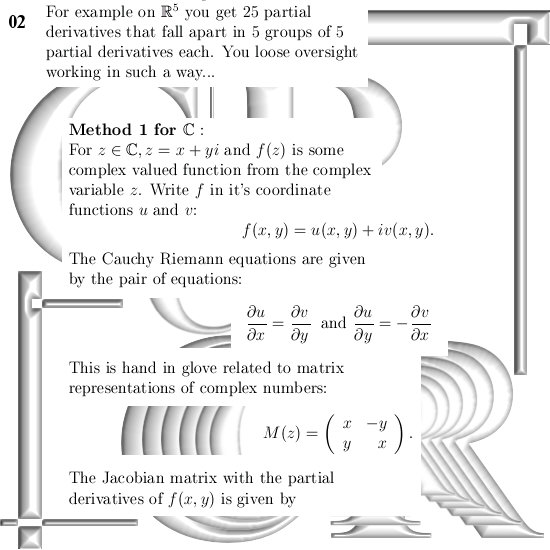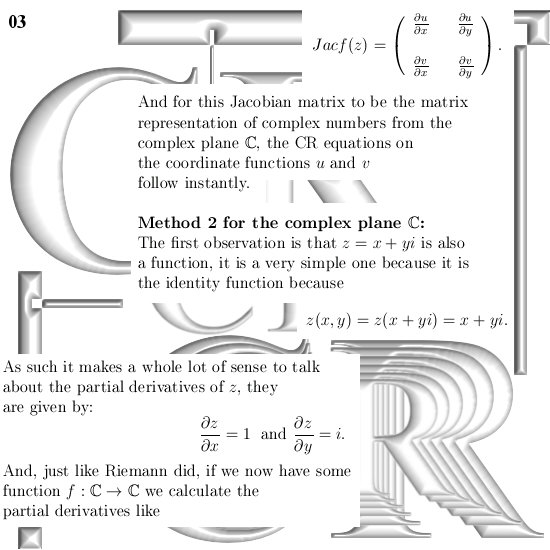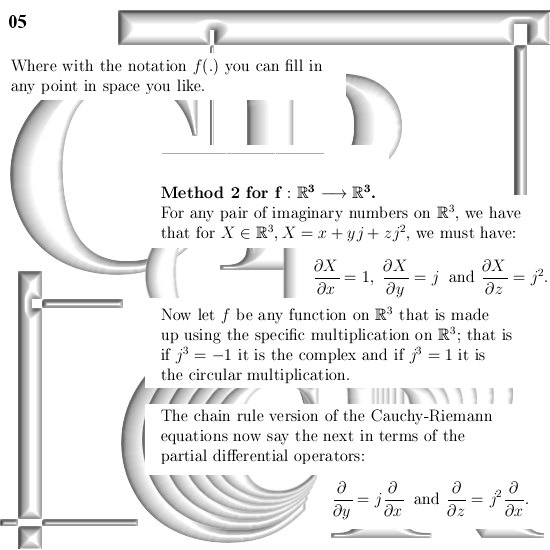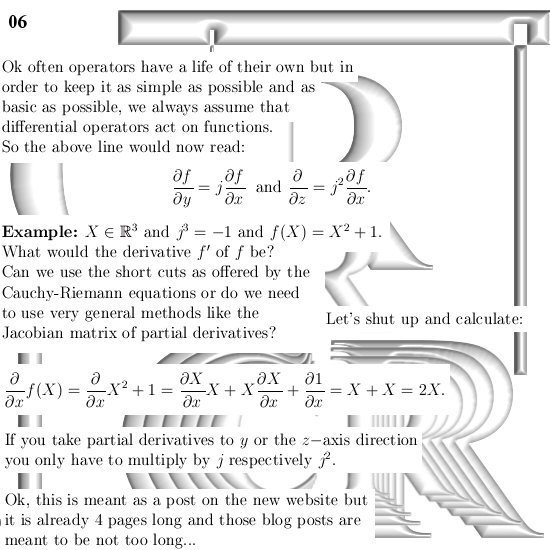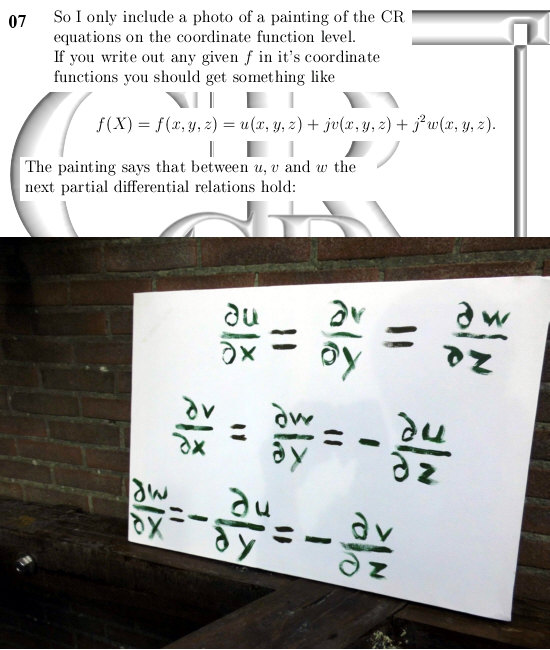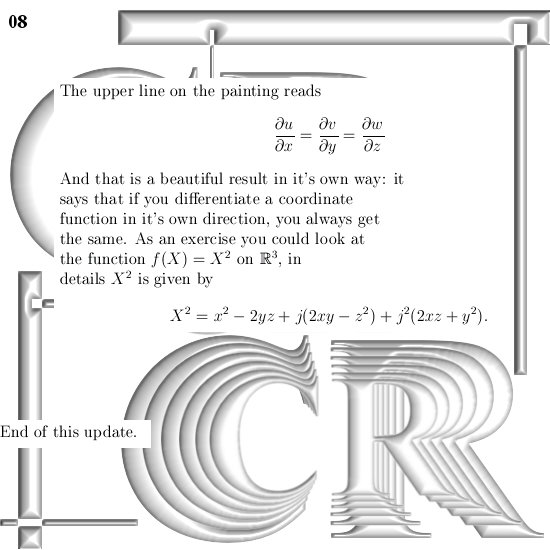This stuff is basic stuff so it should be hanging out on this new website.

Till update my dear reader.